# Simple Math¶

I hope you are having fun working with Scratch. Remember that we are trying to learn concepts here, not just have fun.

Note

I almost feel I am overworking this fun stuff. I know some of you are struggling with all this - and it definitely ain’t fun when that happens. But like me crashing my model airplanes, which was not fun and was even expensive for a poor kid, keeping at it is the only way you will succeed. Eventually it becomes fun. I still crash models, but I have moved on and now fly real airplanes. I have not crashed these yet, even though this is how I fly sometimes:My instructor did spend a lot of time explaining how to get out of the plane if we broke it - we did wear parachutes when flying like this! Yes, it is fun when you know what you are doing!

## Evaluating Expressions¶

We have been doing simple math work without really paying much attention to what was going on. We really need to know a few things about math in the computer, so let’s dig in a bit deeper.

Here is what we want to work with, it should look familiar to you - assuming you remember your math class!

• A + B + C - D.

Now, what I just presented was a sequence of variable names and math operators. The operators are those symbols I use to indicate addition and subtraction above. The entire sequence of things is called an expression and we can ask the computer to evaluate it!

### Parts of an expression¶

Expressions can be constructed out of several kinds of symbols that you get to create as a programmer.

#### Variables¶

You define containers to hold different kinds of data your program will manipulate. We discussed that earlier. In this exercise, we choose names that help us remember what the container holds. Names can be pretty long, or too short to be meaningful, like those I am using in the expression above. We call one of these containers a variable because the value it holds at any moment can vary as your program runs.

In most languages, we declare variables in our programs. Here are some other examples:

```int var1 = 10;
float PI = 3.1415926;
```

The first line asks the system to create a container that can hold a number that has no fractional part - we call it an integer. The second line sets up numbers that can have fractional parts, we call them floating point numbers, because the decimal point can wander around a lot!

Most programming languages come with a variety of templates for containers that hold different kinds of data. Since we are doing math in this lecture, we will just work with these two kinds of containers for now.

Our variables live in the computer’s memory while our program runs. We use the name for the variable when we want to do something with a variable, the computer just looks up where it lives in memory!

Note

Remember how you did division when you were a kid? “How many times does 5 go into 23?” you were asked. The answer was 4 with 3 left over - the remainder. These integer numbers are the kind that work funny until you get used to them. If we are working with integer numbers and we do division, there is no fractional part - what you think should be there is just chopped off and thrown away. 5/2 = 2 and 2 / 5 = 0! This takes getting used to so try to remember this!

#### Literal numbers¶

Our expressions could also contain numbers that we just type into the expression. Each of these numbers is called a literal number since it is literally just sitting there in our expression!

It is important to remember that the computer stores integers using one coding system, and floats using a different coding system. We cannot just mix the two together. The math operators might need to convert one kind of number into the other by figuring out how to move from one code to another. If we convert a float with a fractional part into an integer - we just chop off the fractional part and throw it away.

#### Math operators¶

You know what most of these are. For now, we will stick with the basic four, and the symbols we use for those operations:

• addition (“+”)
• subtraction (“-“)
• multiplication (“*”)
• division (“/”)

(The double quotes are not part of the operator - just the single character).

### Evaluating Expressions¶

Our task for this lecture is to figure out how the computer goes about evaluating expressions.

There is a lot to learn here, but I will try not to get you confused! Here we go:

#### Basically, scan the expression left to right¶

The computer will evaluate an expression by working from left to right through all the symbols it finds (variables, literals, operators) trying to figure out what to do. Unfortunately, the rules of the language may cause us to scan the expression several times before we figure everything out! This is what makes understanding how the computer evaluates expressions harder than it seems like it should be!

#### Examining variables¶

When the system see a variable name in an expression (like we are considering here), it goes into memory, finds the named container, peeks inside and fetches out the value that is there at that moment. The system knows what kind of container it is working with, because you told it that when you declared that container. If it sees the same name again in the expression, it goes back and looks at it again! Something could have happened to that container since it last looked, so it checks again! That makes sense.

#### Examining literal numbers¶

When the processor sees a literal number, it takes the sequence of characters you typed, and converts those characters into the right code to represent the number correctly in your program. It does not put that number anywhere special, it just hangs on to it for use later. That makes sense as well.

As the processor scans the literal number it notes whether that sequence of characters included a decimal point or not. If so, it treats the number as a floating point number, otherwise it treats it as an integer number. Our expression can give different answers depending on what the various kinds of numbers are! Yikes! (we will work through this later.)

OK, what is the catch? This seems pretty easy! Well, now we need to look at those operator gadgets!

#### How the operators work¶

There is a simple way to show how computers do things. That way involves a simple diagram called a “railroad diagram”. We call it that because it looks like the model railroad tracks you probably saw as a kid. The computer follows these “tracks” looking for things that control which way it moves. . Hopefully, these diagrams will make sense, without getting bogged down in the details here.

So, how do I know what the computer will do when it tries to evaluate that funny expression we had up above.

#### Start with just addition¶

We need to see how the system will process a simple expression, so let’s build a simple diagram and see what we get: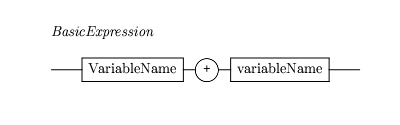Here, we have a rule that allows for a basic expression just like the one shown above, only shorter. The boxes named VariableName live on either side of the addition operator (“+”). Now, evaluating this expression is pretty easy.

Now, as we discussed last time, the system processes this expression by starting at the left side of the diagram and looking at the symbols it sees. When it gets to the box named variableName it looks at your program to see what name is there, then goes into memory to see what value is currently stored in that named container. When it sees the addition operator it remembers it for a moment, then gets the next piece (another variableName here). Now it has the three things it needs to do the work. The two things to combine, and the operator to use. It performs the math, leaving a single value. In this example the expression has been fully evaluated and we are left with the value.

Life gets a bit more complex if we extend this example.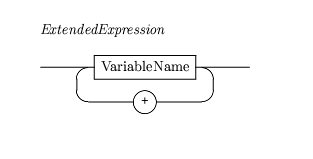Here the diagram says we can have as many variables in the expression as we like, and place the addition operator between each pair. Now, the evaluation works like this:

Start at the left side of the diagram. Enter the variableName box, and check to see what variable you are working with in your program. Go get that value and remember it. Next, see if there is an addition operator. If so, remember that and continue, we enter the variableName box again, and we look at our program to see what the next variable is. We fetch the value of that variable, and, using the addition operator we add the second value to the first value and remember this new number.

Now, we check to see if there is another adding operator, if so, we loop around again and add in the next part. You should see that this will slowly add together all the parts.

How do we know when to stop spinning? When we have processed our entire expression and have not seen yet another adding operator we know we are done!

Now that does not seem so complicated, does it.

#### What about subtraction?¶

We can modify this last diagram in a simple way and see how to handle expressions that mix both additions and subtractions. Here is what it looks like: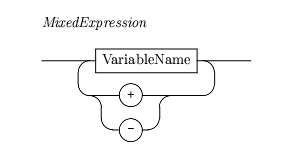Now, we will allow either of these operators to keep us in the loop. We will note which operator we see and use it when we figure out the next value to work with. Thus, we will either add or subtract that next value (after the operator) from the value we came up with as we processed the expression up to the current operator. Try a few simple examples and verify that you can see what will happen (we will do more of this in the next lab).

Now for the hard part.

#### What about multiplication and division?¶

Now we have a basic problem. What do you think I might get if I try to evaluate these expressions?

```A * B - D + D / E
A - B * C + E / F
```

Now, we could just evaluate the expressions from left to right, but that is not how things are done in computer-land. Instead, the designers of programming languages like C++ assign something called a precedence level to each operator, and it is that precedence level that controls how an expression is evaluated.

#### Operator Precedence¶

Basically, it works like this:

Multiplication and division are considered more important (have higher precedence) than addition and subtraction. So, when we scan from left to right, we search for the highest precedence operator we can find, and do that operation first. When we perform this, we peek at the variables (or literals) on either side of the operator, get the values and do the operation. This effectively eliminates that small part of the expression and replaces it with a single number. Then we rescan again looking for the highest precedence operator we have not handled yet, and so on. In the end, we will have done any multiplications or divisions we find first, then do the add and subtract stuff later.

Perhaps seeing the railroad diagrams for this will help: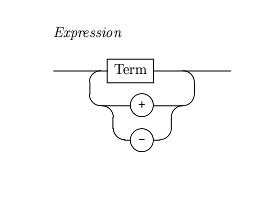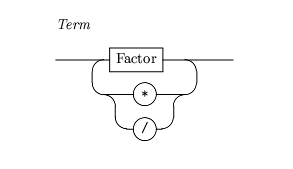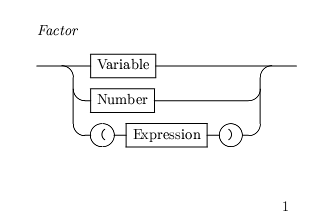Now, do not get overwhelmed by these diagrams. They are just one way to help you see what the computer is doing as it processes an expression. If that way does not help you, perhaps we can clear things up with that other technique we will get to in a moment.

### Let’s see how the diagrams work.¶

We start off on the diagram named Expression and start looking at our real expression. We see that we need to get something called a Term first, so we head off to that diagram and follow it until we are done.

The Term diagram says we first need to find something called a Factor, so we head off once again - boy this seems silly!

The Factor diagram says we could see a VariableName next, which is what we have in our expression. According to the diagram, that is all we need to complete the diagram for Factor, so we wander back to the Term diagram. Here is where the going gets a bit tough.

We are just following the diagrams along, but we are looking at the symbols in our expression. If we see a multiply (or divide) operator in our expression, the Term diagram says we go back down to the Factor diagram and see what comes next. If we do not see one of these symbols, we must be done with the Term diagram, so we go back up to our Expression diagram and see if the next symbol either the addition or subtraction operator. If we find one of those, we head back down once again and keep looking at our symbols.

Only when we get our second variable value (the one that is on the right side of an operator) do we do the math. This can be a little hard to see, so let’s work an example and see how it all works.

• A + B * C

Now, I am going to list the diagram we are in, and the symbol we are looking at, where we go next, and try to show when we do the math in a table. Here goes:

 Symbol Current Diagram Next Diagram Operation A Expression Term (none) A Term Factor (none) A Factor Term look up value for A and remember it “+” Term Expression (none) “+” Expression Term remember the addition operator B Term Factor (none) B Factor Term look up value for B and remember it “*” Term Factor (none) C Factor Term look up value for C, multiply by value for B Term Expression take last result and add it to value for A

If you followed all of this, you see that the multiplication happened before the addition - exactly as the rules said it should.

Believe it or not, the folks who write the tools that process your program use diagrams like this to think their code through - and that is why it helps to understand how the diagrams work - they really do explain how the language works!

#### What about literals¶

If you look at that Factor diagram, you see that we can include literal numbers in our expressions. That makes sense! The system just converts the literal number into a value and uses that value just like it uses a value it found in the variable container when we included a variable name in the expression.

There is a bit of a puzzle in how the system handles a mixture of integer and floating point values. Basically, what it does is to look at both values it is about to use with an operator. If both are integer values then integer math will be done (no fractions, remember?). If either number is a floating point number, both are converted to floating point and floating point math is done. This can get confusing! (Watch out for examples of this on a test!)

Try these out and see if you get the right answer:

```5 / 2           // should give 2
5.0 / 2         // should give 2.5
5 / 2.0         // should give 2.5
2 / 3           // should give 0
3 / 2 * 3       // should give 3
1 / 3 * 3       // should give 0
1.0 / 3 * 3     // should give 1, but does not (more on this later)
1.0 / 3.0 * 3.0 // should give the same value as above
```

#### What about those parentheses?¶

It seems we can put parentheses around sub-expressions if we like. What does this do? Well, if you place some part of the expression inside parentheses, you essentially force the system to pause in evaluating the expression you started with and start over, working on the smaller expression inside the parentheses. When that smaller expression is fully evaluated, that single value can be combined with other things going on in the outer expression.

Phew! But it makes sense and that is just what you used to do in math class and with your calculator!

Notice that we can have parenthesized subexpressions inside other parenthesized subexpressions. It is common to mess This up and not have the right number of open and close parentheses as expressions get more complicated. Programmers learn to count the open and close parentheses to make sure the expression is valid (and then check that the expression is really what they want!)

### Working through the expression another way¶

As I said earlier, if the diagrams prove too confusing, there is another way to work out what will happen. Let’s try that now.

Here is our starting point:

• A * B - D + D / E

We will be scanning from left to right, searching for the highest precedence operator in the expression. According to our rules, the highest precedence is either multiplication or division, so the first operator we find is the multiplication between A and B. Let’s put parentheses around that part of the expression. Like so:

• (A * B) - D + D / E

Once we have parentheses around this part, we will not scan inside the parentheses again, pretend the system has reduced this part to a single value.

Now start scanning again. The next highest precedence operator we see is the division. Place parentheses around that part and we get this:

• (A * B) - D + ( D / E)

Now, we have run out of multiplications and divisions, we scan again looking for additions and subtractions. The first one we find is the subtraction. Place parentheses around the two parts that get subtracted. The left side of that operator is that stuff we multiplied first. Here is where the parentheses go this time:

• ( ( A * B ) - D) + ( D / E )

Last pass will finish off the addition and we place parentheses around both parts that get added and we get this:

• ( ( ( A * B ) - D ) + ( D / E ) )

This last expression is called “fully parenthesized” (boy is it full of parentheses!) You have used parentheses in math before to group parts of the expression so you can see what is really happening. If you have a Texas Instrument calculator, you use the parentheses keys to set up the calculation. That is exactly what we are doing here.

It seems complicated, but with a little practice, you do not even think about it.

Do not be afraid to add parentheses to make sure the expression works the way you expect it to!

### One more funny operator¶

There is another operator we need to examine. It is called the Modulus operator, and it has the same precedence as multiply and divide. Before we can understand what happens in this operator, we need to discuss how integer math works in a bit more detail

#### What is that you say, you know how math works.¶

Well, remember that our computers are not capable of doing the kind of math you are probably used to. They do funny math - discrete math!

#### What is the result of dividing 5 by 2?¶

Now, those of you did not remember our earlier discussion about this and said 2.5, remember that we are in the computer’s world. The answer in this case is just 2! Why. Because the two numbers I presented were integer numbers and when we divide two integers we throw away any fractional part. We do not round, we just toss!

Now, let’s ask a different question. What is left over if I take out as many 2’s as will fit completely into 5? Hmmm, let’s see I can get two 2’s out of 5 before I have a problem. When I finish taking those 2’s out I have 1 left over.

That is what you get when you use the modulus operator - the symbol for which is a percent sign.

• 5 % 2 = 1

Now what on earth would we use this for?

Suppose I am creating a long report, with thousands of lines of text. Every 77 lines, I want to make the printer move to a new page. How could I do that.

Well, if I set up a line counter to track how many lines I have displayed, then I could use an expression like lineCount % 77 and see if the value of that expression is zero. If so, I have hit a count evenly divisible by 77 (no remainder) and I should skip to a new page.

We will see how this is done later in the course.

# What about that 1.0/3.0*3.0 thing?¶

There is one more funny thing about computers. You just `know` that an expression like 1.0/3.0 * 3.0 is 1.0, right? Well, computers cannot deal with things like 1.0/3.0. You know that as 0.3333333(to an infinity of 3’s). Well the computer cannot store an infinity of anything - no matter how much memory you have, so it chops off the sequence at some point. This generates a real error in the calculation, but a small one. Now if we multiply that by 3.0, we get something like 0.999999999995, which is almost right, but still wrong. All of this is why we study `Discrete Math` in detail to figure out how to deal with these errors in our programs. Fortunately for you, this kind of math is not something you see until your senior year in college, or in graduate school!